Question

# As shown in the cireuit to the right, a potential difference of 625 V is applied...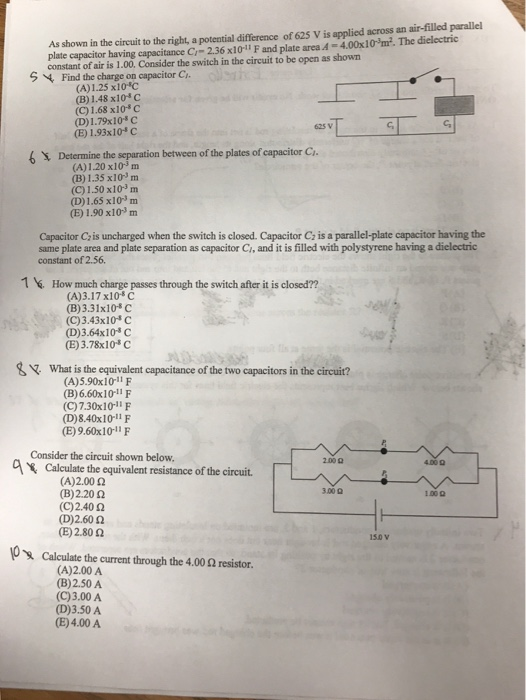As shown in the cireuit to the right, a potential difference of 625 V is applied across an air-filled parallel plate capacitor having capacitance Cr-2.36 x101l F and plate area 4-4.00x10m2. The dielectric constant of air is 1.00, Consider the switch in the circuit to be open as shown S 4 Find the charge on capacitor C (A)1.25 x10*C (B) 1.48 x10 c (C) 1.68x10 (D) 1.73x10-C (E) 1.93x10*c 625 v G, 6 Determine the between of the plates of capacitor Ci (A)1.20 x103m (B) 1.35 x103 m (C) 1.50 x103 m (D)1.65 x10m (E) 1.90 x10m Capacitor Cis uncharged when the switch is closed. Capacitor Cz is a parallel-plate capacitor having the same plate area and plate separation as capacitor Ci, and it is filled with polystyrene having a dielectric constant of 2.56. 1 6. How much charge passes through the switch after it is closed?? (A)3.17 x108 C (B)3.31x10 C (C)3.43x10*C (D)3.64x10* C (E)3.78x103 C What is the equivalent capacitance of the two capacitors in the circuit? (A)5.90x10-11 F (B)6.60x10-11 F (C)7.30x1011 F (D)8.40x10-11 F (B) 9.60x10-1 F Consider the circuit shown below. 200 0 Calculate the equivalent resistance of the circuit. (A)2.00 2 (B)2.20 2 (C) 2.40 ? (D)260 ? (E) 2.30 ? 3.00 0 1.00? 15.0 v Calculate the current through the 4.00 ? resistor. (A)2.00 A (B)2.50 A (C)3.00 A (D)3.50 A (E)4.00 A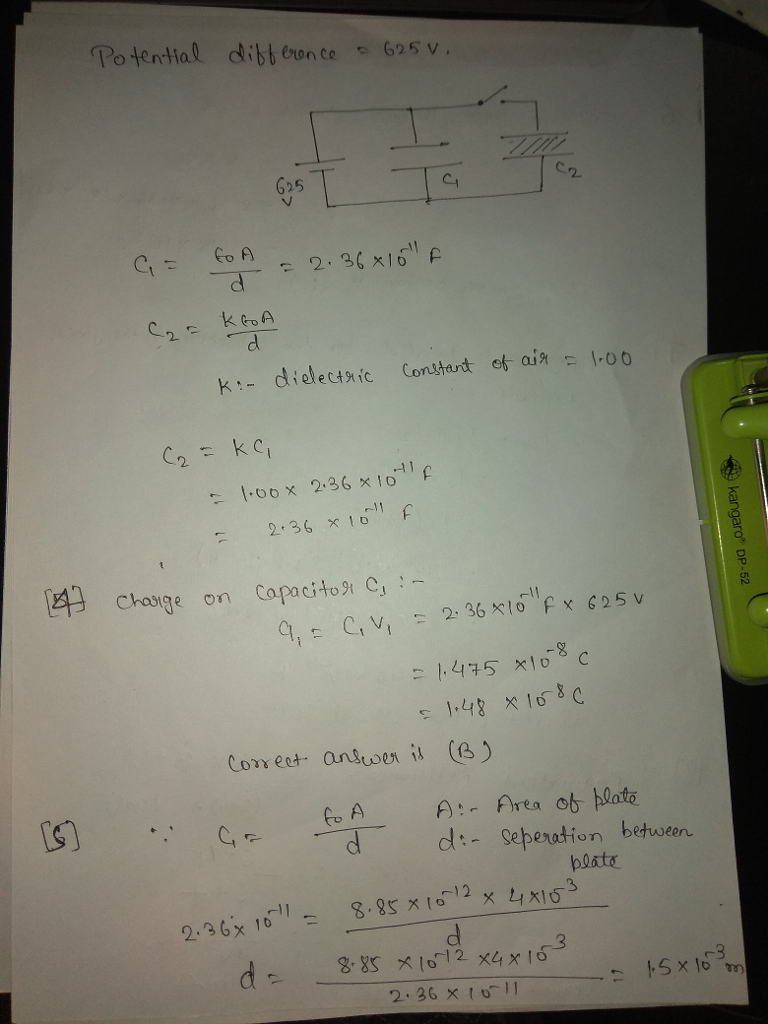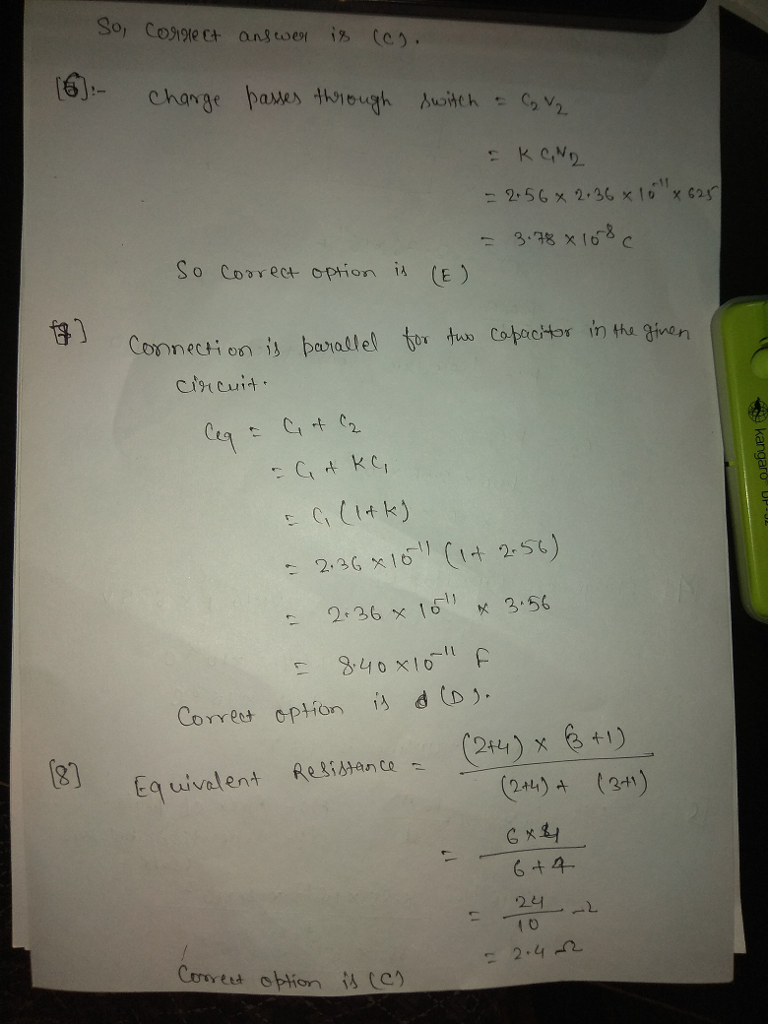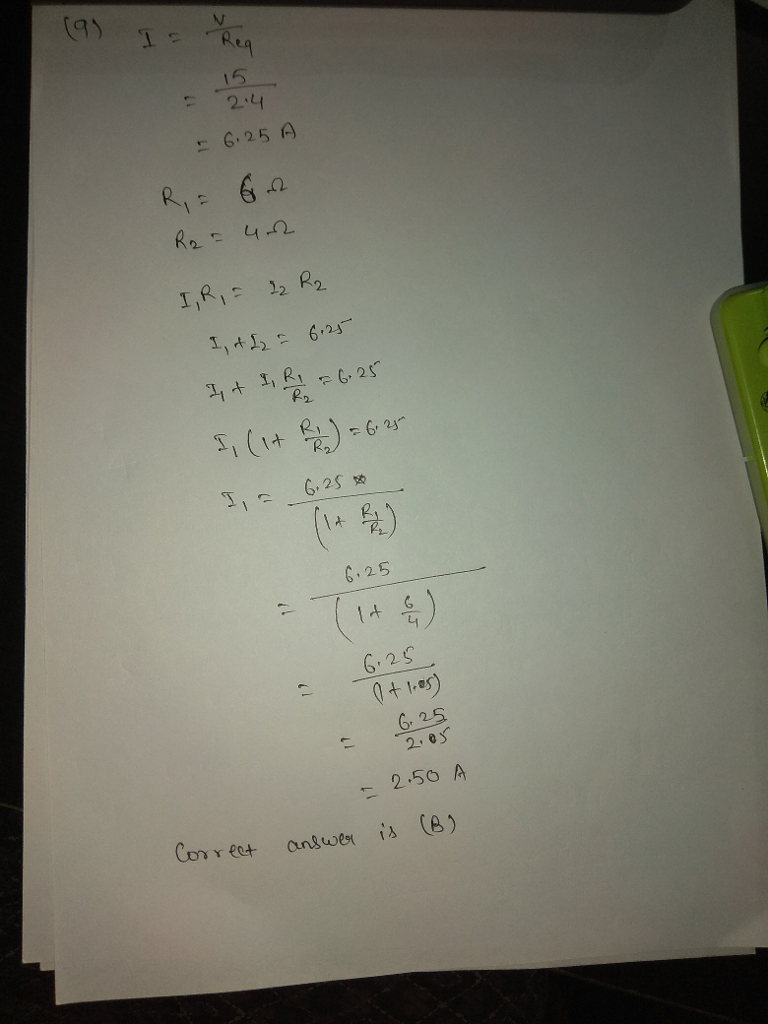#### Earn Coins

Coins can be redeemed for fabulous gifts.

Similar Homework Help Questions
• ### Problem 1 Consider the following circuit with four fully charged parallel-plate capacitors V= 20.0 V Figure...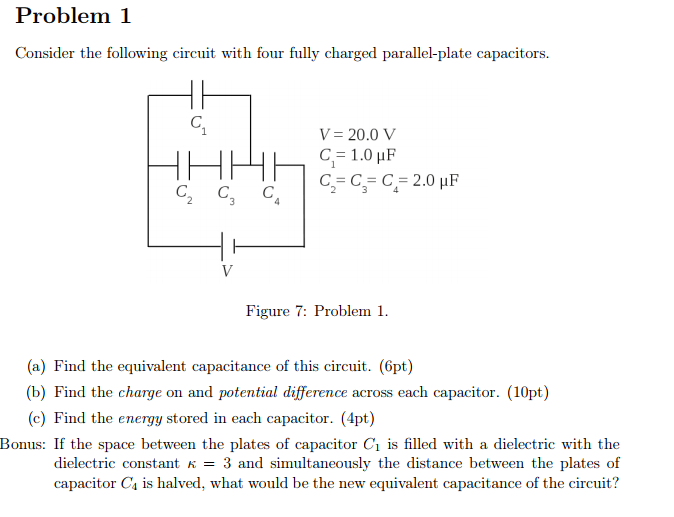Problem 1 Consider the following circuit with four fully charged parallel-plate capacitors V= 20.0 V Figure 7: Problem . (a) Find the equivalent capacitance of this circuit. (6pt) (b) Find the charge on and potential difference across each capacitor. (10pt) (c) Find the energy stored in each capacitor. (4pt) Bonus: If the space between the plates of capacitor C1 is filled with a dielectric with the dielectric constant K = 3 and simultaneously the distance between the plates of capacitor...

• ### (a) State the units of electric potential and electric potential energy. Sketch an equipotential ...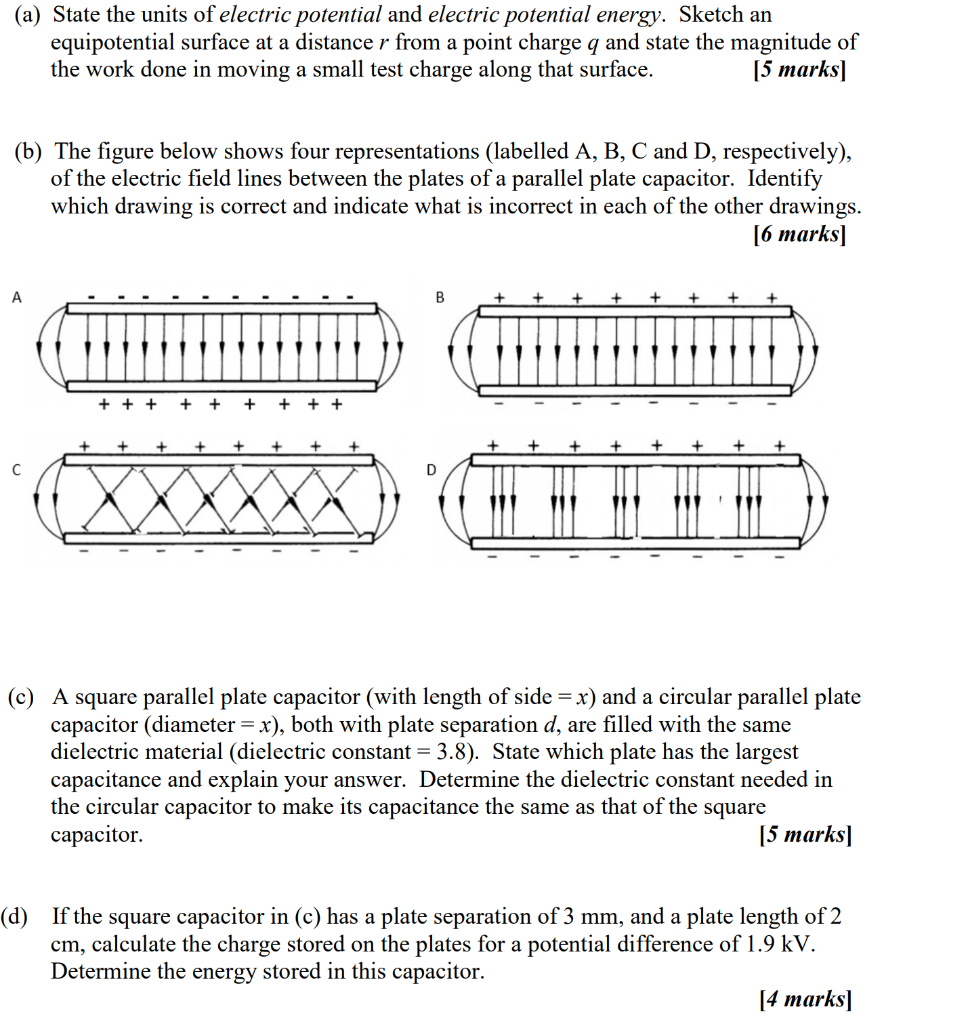(a) State the units of electric potential and electric potential energy. Sketch an equipotential surface at a distance r from a point charge q and state the magnitude of the work done in moving a small test charge along that surface. [5 marks] (b) The figure below shows four representations (labelled A, B, C and D, respectively), of the electric field lines between the plates of a parallel plate capacitor. Identify which drawing is correct and indicate what is incorrect...

• ### 20 volts are applied to a parallel-plate capacitor with a distance of 1 mm separating two...

20 volts are applied to a parallel-plate capacitor with a distance of 1 mm separating two electrodes and with a dielectric material having e_r= 120. The surface area of each plate is 1cm^2 and e_0= 8.85 x 10^-12 Fm^-1. Please calculate (1 F = 1 C/V): (a) the total charge density (b) the bound charge density (c) the polarization (d) the permittivity of the dielectric (e) the electric displacement (f) electric susceptibility (g) the capacitance

• ### Two parallel plates, each having area A 3676cm are connected to the terminals of a battery of voltage V, 6 V A as shown...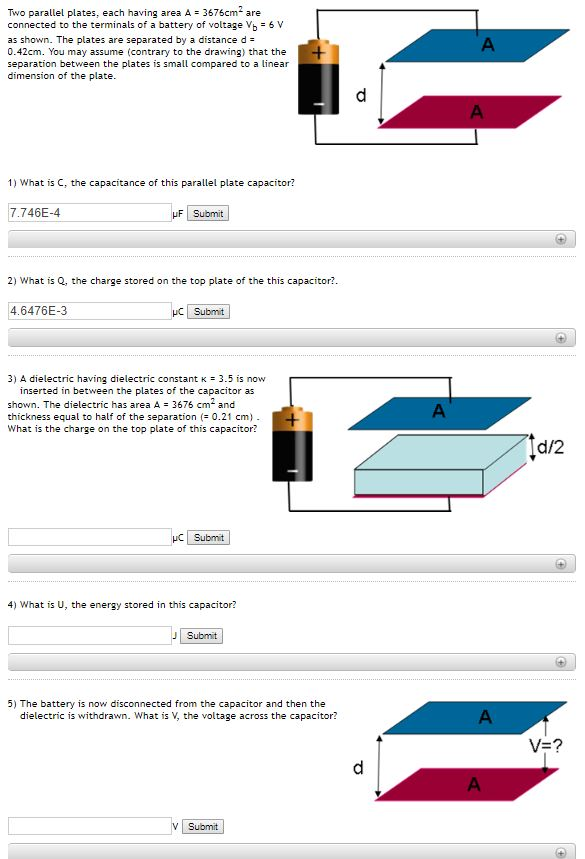Two parallel plates, each having area A 3676cm are connected to the terminals of a battery of voltage V, 6 V A as shown. The plates are separated by a distance d 0.42cm. You may assume (contrary to the drawing) that the separation between the plates is small compared to a linear dimension of the plate + A 1) What is C, the capacitance of this parallel plate capacitor? 7.746E-4 uF Submit 2) What is Q, the charge stored on...

• ### Task 3 a) The capacitor having a capacitance of 10 uF is applied with a potential...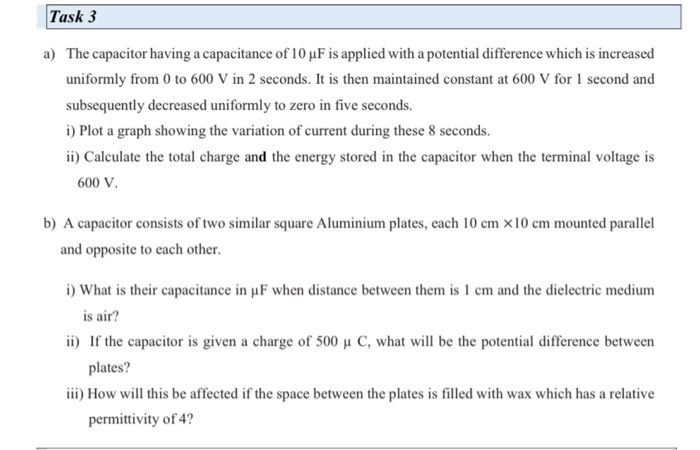Task 3 a) The capacitor having a capacitance of 10 uF is applied with a potential difference which is increased uniformly from 0 to 600 V in 2 seconds. It is then maintained constant at 600 V for 1 second and subsequently decreased uniformly to zero in five seconds. 1) Plot a graph showing the variation of current during these 8 seconds. ii) Calculate the total charge and the energy stored in the capacitor when the terminal voltage is 600...

• ### A parallel-plate capacitor is filled with a dielectric with K = 4. The plate area is 3 m2 and...

A parallel-plate capacitor is filled with a dielectric with K = 4. The plate area is 3 m2 and the plate separation is 2 mm. The capacitance of this capacitor isa) 210 nF.b) 18 nF.c) 8 nF.d) 13 nF.e) 53 nF.

• ### Problem 5 In the figure below C1 2.0 μF, G 10.0pE, C3 3,0 μF , C.-6.0 μF.G-4.0 μ. The applied potential difference between a and b is Vab 10V. a) [4 points] Calculate the equivalent capacitance o...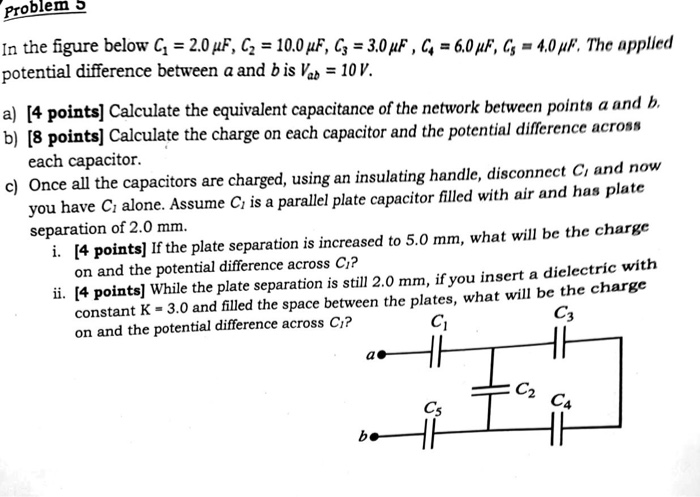Problem 5 In the figure below C1 2.0 μF, G 10.0pE, C3 3,0 μF , C.-6.0 μF.G-4.0 μ. The applied potential difference between a and b is Vab 10V. a) [4 points] Calculate the equivalent capacitance of the network between points a and b. b) [8 pointsl Calculate the charge on each capacitor and the potential difference across each capacitor. c) Once all the capacitors are charged, using an insulating handle, disconnect Ci and now you have C alone. Assume...

• ### Part (A) Find the equivalent capacitance of the capacitors in the figure below. (10 points) 6,00...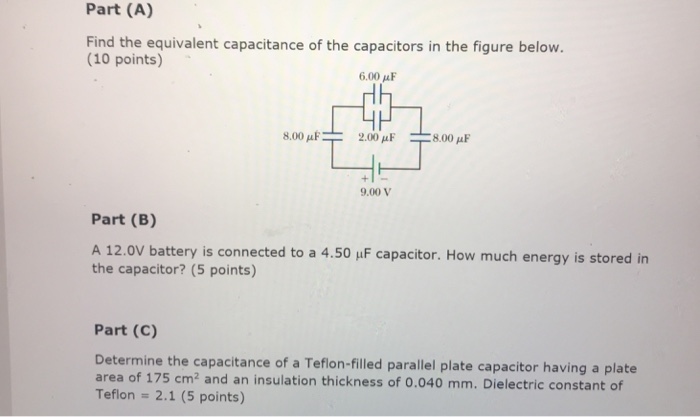Part (A) Find the equivalent capacitance of the capacitors in the figure below. (10 points) 6,00 μF Hh 8.00 μ F 2.00 μF 8.00 μF 9.00 V Part (B) A 12.0V battery is connected to a 4.50 uF capacitor. How much energy is stored in the capacitor? (5 points) Part (C) Determine the capacitance of a Teflon-filled parallel plate capacitor having a plate area of 175 cm2 and an insulation thickness of 0.040 mm. Dielectric constant of Teflon - 2.1...

• ### COI) (15 pts) Two capacitors, C and C2, are connected in series to a 60 V battery as shown in the sketch in part b). The capacitance of C, is 1.0 x10 F, and it carries a charge of2.0 10 C. R-100 ohms...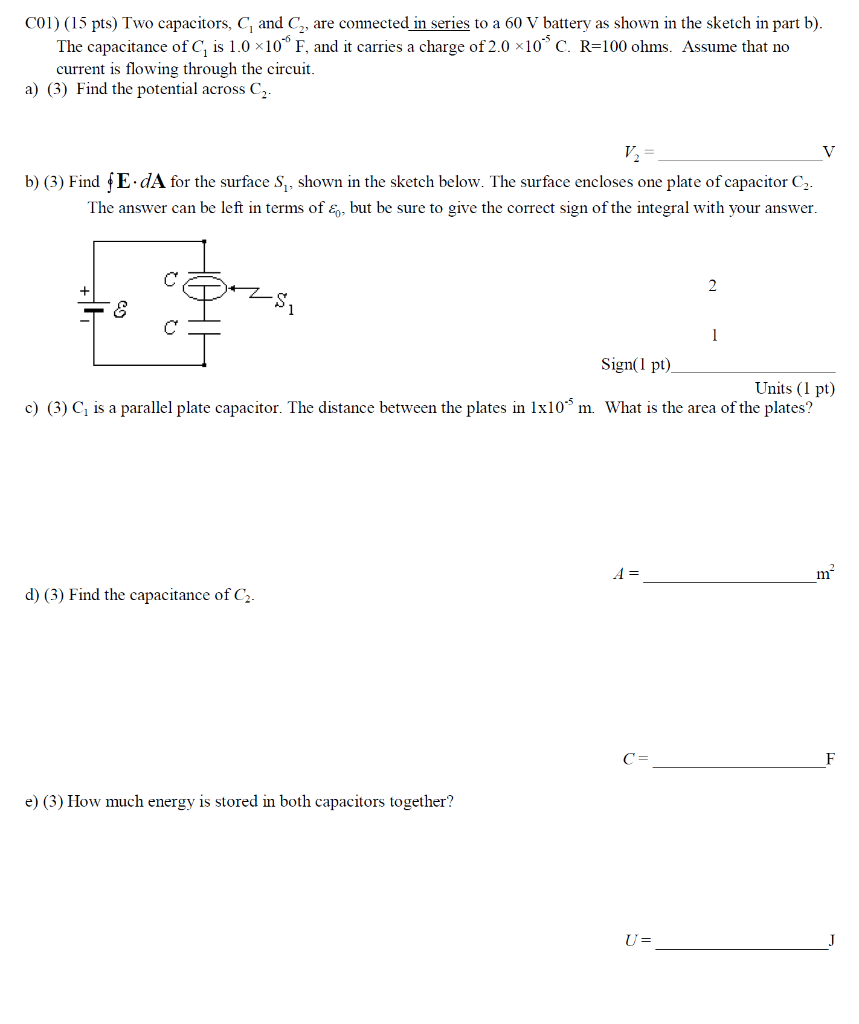COI) (15 pts) Two capacitors, C and C2, are connected in series to a 60 V battery as shown in the sketch in part b). The capacitance of C, is 1.0 x10 F, and it carries a charge of2.0 10 C. R-100 ohms. Assume that no current is flowing through the circuit. a) () Find the potential across C b) (3) Find fE dA for the surface S, shown in the sketch below. The surface encloses one plate of capacitor...

• ### The figure shows a parallel-plate capacitor of plate area A and plate separation d. A potential differenceV0 is applied between the plates.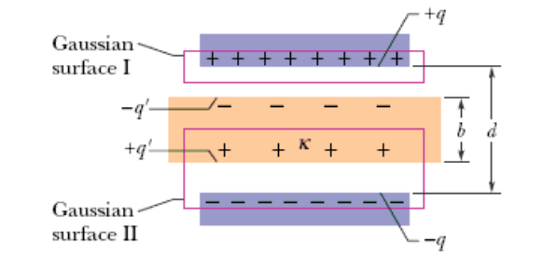The figure shows a parallel-plate capacitor of plate area A and plate separation d. A potential differenceV0 is applied between the plates. While the battery remains connected, a dielectric slab of thickness b and dielectric constant κ is placed between the plates as shown. Assume A = 130 cm2, d = 1.94 cm, V0 = 72.6 V, b = 0.735 cm, and κ = 3.15. Calculate (a) the capacitance,(b) the charge on the capacitor plates,(c) the electric field in the gap, and(d)...